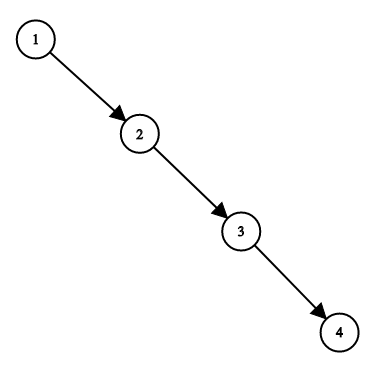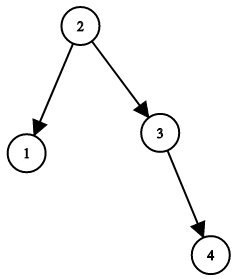## 1382. Balance a Binary Search Tree

Given a binary search tree, return a balanced binary search tree with the same node values.

A binary search tree is balanced if and only if the depth of the two subtrees of every node never differ by more than 1.

If there is more than one answer, return any of them.

Example 1:```Input: root = [1,null,2,null,3,null,4,null,null]
Output: [2,1,3,null,null,null,4]
Explanation: This is not the only correct answer, [3,1,4,null,2,null,null] is also correct.
```

Constraints:

• The number of nodes in the tree is between `1` and `10^4`.
• The tree nodes will have distinct values between `1` and `10^5`.

## Rust Solution

``````struct Solution;
use rustgym_util::*;

trait Inorder {
fn inorder(self, nodes: &mut Vec<i32>);
}

fn inorder(self, nodes: &mut Vec<i32>) {
if let Some(node) = self {
let mut node = node.borrow_mut();
let val = node.val;
let left = node.left.take();
let right = node.right.take();
left.inorder(nodes);
nodes.push(val);
right.inorder(nodes);
}
}
fn build(nodes: &[i32]) -> TreeLink {
let n = nodes.len();
if n == 0 {
None
} else {
let m = n / 2;
tree!(
nodes[m],
)
}
}
}

impl Solution {
let mut nodes: Vec<i32> = vec![];
root.inorder(&mut nodes);